## 颗粒群碰撞搜索及CFD-DEM耦合分域 求解的推进算法研究1)

*东北石油大学机械科学与工程学院, 黑龙江大庆 163318

## RESEARCH ON PARTICLE SWARM COLLISION SEARCH AND ADVANCEMENT ALGORITHM FOR CFD-DEM COUPLING DOMAIN SOLVING1)

Liu Jubao*, Wang Ming,*,2), Wang Xuefei*, Yao Liming, Yang Ming,*,3), Yue Qianbei*

*School of Mechanical Science and Engineering, Northeast Petroleum University, Daqing 163318, Heilongjiang, China

School of Mechanical and Aerospace Engineering, Nanyang Technological University, 639798, Singapore

 基金资助: 1)国家自然科学基金资助项目.  11972114国家自然科学基金资助项目.  51904075

Received: 2021-01-1   Accepted: 2021-03-19   Online: 2021-06-18Abstract

When the computational fluid dynamics discrete element method (CFD-DEM) is used for solid-liquid two-phase coupling analysis, the selection of particle calculation time step directly affects the accuracy and efficiency of the coupling calculation. For this reason, each target particle is selected as the research object, and interpolation function is introduced to calculate the motion displacement of the time step, and a variable spatial search grid is constructed. An improved particle collision search algorithm (modified discrete element method, MDEM) was proposed by selecting possible collision particles to build a search list and using reverse search Method to judge collision particles. The algorithm in particle group and fluid coupling calculation, the particle counting the initial time step selection particle collision time without limit, realization of automatic adjustment and correction by large step, calculated by the real-time update of fluid particles and fluid coupling conditions, time step, the granular computing time step selection, as a result of low computational efficiency, selection is too large too small to solve the problem of false negatives, particle collision of particles and fluid coupling numerical simulation provides a effective calculation method. Through the numerical simulation of two particles and multiple particles, the relative errors of the collision forces, collision positions and times between particles obtained are all less than 2% compared with the theoretical calculation results. Compared with the traditional DEM collision search algorithm, the three calculation time steps selected do not affect the calculation accuracy, and the calculation efficiency is higher. Through the coupling numerical simulation of multiple particles and fluid, using the traditional CFD-DEM method, the precise solution can be obtained only when the particle calculation time step is 10$^{-6}$ s or smaller, while the precise solution can be obtained by using the proposed method to take 10$^{-4}$ s, which avoids the problem of missed decision caused by particle collision with the increase of time step, and the calculation time is reduced by 16.7%.

Keywords： two-phase flow ; collision search algorithm ; CFD-DEM ; coupled calculation method

Liu Jubao, Wang Ming, Wang Xuefei, Yao Liming, Yang Ming, Yue Qianbei. RESEARCH ON PARTICLE SWARM COLLISION SEARCH AND ADVANCEMENT ALGORITHM FOR CFD-DEM COUPLING DOMAIN SOLVING1). Chinese Journal of Theoretical and Applied Mechanics, 2021, 53(6): 1569-1585 DOI:10.6052/0459-1879-21-002

## 引言

Baraff提出了边界盒算法, 使用轴向对齐的边界盒来确定颗粒之间的碰撞. 如果两个颗粒相撞, 其在$x$, $y$和$z$维度上的正交投影将会重叠. 3个列表包含对轴的投影坐标, 每个维度对应一个列表. 在下一个时间步骤, 需要再次对之前排序的列表进行排序. Li等提出的时间相干碰撞搜索算法 (SMB), 是将包围盒AABB投影在笛卡尔坐标轴上, 根据投影间距递增排序建立初步碰撞搜索列表, 通过对列表内所有间距搜索检查确定是否重叠, 建立碰撞列表. 该算法适用于大量移动AABB的系统, 并支持对象的动态插入和删除, 不受物体大小的限制.

## 1 流体与颗粒群耦合的数值分析方法

### 图1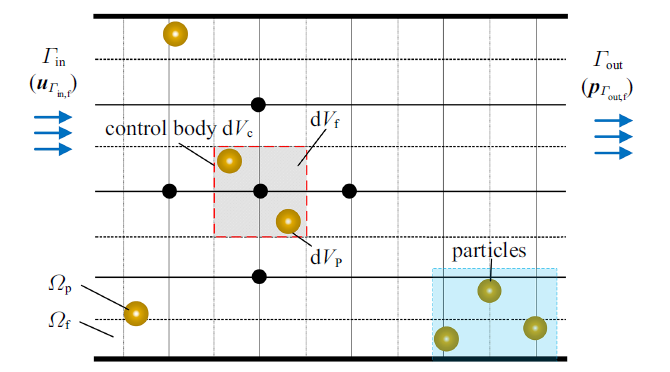Fig.1   Schematic diagram of fluid and particle group coupling model

### 1.1 流体控制方程

$\dfrac{\partial (\alpha_f \rho _f )}{\partial t}+\nabla \cdot \rho _f \alpha_f { u}_f =0$

$\dfrac{\partial }{\partial t}\left( {\alpha_f \rho _f { u}_f } \right)+\nabla \cdot \left( {\alpha_f \rho _f { u}_f { u}_f } \right)= \\ -\alpha_f \nabla p_f +\alpha_f \nabla \cdot \overline {\overline {\tau}} + { S}_f +\alpha_f \rho _f { g}$

$\alpha_f =1-\alpha_p =1-\dfrac{1}{V_E}\sum_{i=1}^{n_p } {V_p^{\left( i \right)} }$
${ S}_f =\dfrac{1}{V_E }({ F}_E +{ M}_E)=\dfrac{1}{V_E }\left[ {\sum_{i=1}^{n_p } {({ F}_f^{(i)}+{ F}_f^{(i)} \times H)} } \right]$

### 图2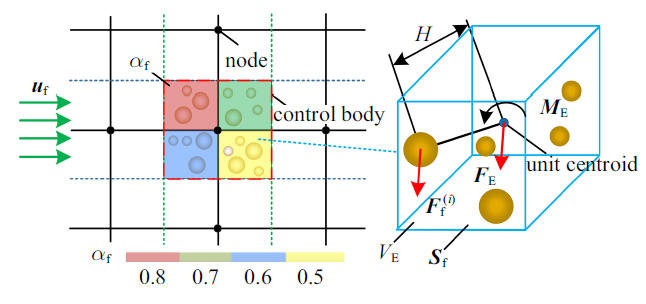Fig.2   Schematic diagram of two-phase fluid calculation unit

### 1.2 颗粒受力分析及动力学方程

1.2.1 颗粒与流体作用力分析

${ F}_f ={ F}_d +{ F}_p +{ F}_vm +{ F}_saff +{ F}_ml +{ F}_b$

${ F}_d =\dfrac{1}{8}C_d \pi \rho _f D_p^{2} \left| {{ u}_f -{ u}_p } \right|({ u}_f -{ u}_p )$
$C_d =\left\{ {\begin{array}{ll} \dfrac{24}{Re_p }(1+0.15Re_p^{0.687}), & Re_p <1000 \\ 0.44, & Re_p \geqslant 1000 \\ \end{array}} \right.$
$Re_p =\dfrac{\alpha_f \rho _f D_p \left| {{ u}_{f} -{ u}_p } \right|}{\mu_f}$

1.2.2 颗粒动力学方程

$m_{i} \dfrac{d{ u}_{i} }{dt}=({ F}_{cn, ij} +{ F}_{ct, ij} )+{ F}_{f, i} +{ F}_{g, i}$
$I_{i} \dfrac{d{ \omega }_{i} }{dt}={ M}_c$

${ M}_c =\dfrac{D_p^{i} }{2}{ F}_{ct, ij}$

1.2.3 颗粒运动轨迹

${ u}_{(t+\Delta t)} ={ u}_{(t)} +(1-\gamma ){ a}_{\left( t \right)} \Delta t+\gamma { a}_{(t+\Delta t)} \Delta t, \ 0\leqslant \gamma \leqslant 1$

${ s}_{(t+\Delta t)} ={ s}_{(t)} +{ u}_{(t)} \Delta t+(1-\gamma ){ a}_{(t)} \dfrac{\Delta t^{2}}{2}+\gamma { a}_{(t+\Delta t)} \dfrac{\Delta t^{2}}{2}$

$\left. {\begin{array}{l} x_{\left( {t+\Delta t} \right)} =x_{\left( t \right)} +\Delta t\left( {u_{\left( {t+\Delta t} \right)}^{x} } \right) \\ y_{\left( {t+\Delta t} \right)} =y_{\left( t \right)} +\Delta t\left( {u_{\left( {t+\Delta t} \right)}^{y} } \right) \\ z_{\left( {t+\Delta t} \right)} =z_{\left( t \right)} +\Delta t\left( {u_{\left( {t+\Delta t} \right)}^{z} } \right) \\ \end{array}} \right\}$

### 1.3 颗粒碰撞模型

$-m_{i} \left( {{ {u}'}_{i} -{ u}_{i} } \right)= m_{j} \left( {{ {u}'}_{j} -{ u}_{j} } \right)={ J}$
$\left. \begin{array}{l} I_{i} \left( {{ {\omega }'}_{i} -{ \omega }_{i} } \right)=\dfrac{D_p^{i} }{2}\left( {{ k}\times { J}} \right)\\ -I_{j} \left( {{ {\omega }'}_{j} -{ \omega }_{j} } \right)=\dfrac{D_p^{j} }{2}\left( {{ k}\times { J}} \right) \end{array} \right\}$

### 图3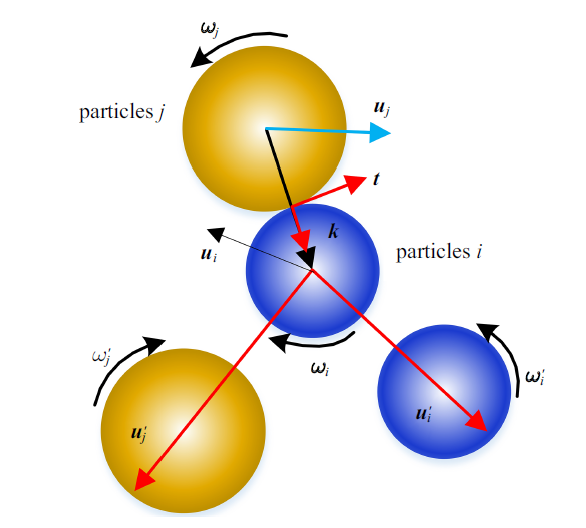Fig.3   Schematic diagram of particle collision

${ {u}'}_{i} ={ u}_{i} -({ k}+\lambda { t})\left( {1+e} \right)\left( {{ k}\cdot { u}_{ij} } \right)\dfrac{1}{1+q}$
${ {u}'}_{j} ={ u}_{j} -({ k}+\lambda { t})\left( {1+e} \right)\left( {{ k}\cdot { u}_{ij} } \right)\dfrac{q}{1+q}$
${ {\omega }'}_{i} ={ \omega }_{i} +\dfrac{5}{D_p^{i} }\left( {1+e} \right)\left( {{ k}\times { \tau }} \right)\left( {{ k}\cdot { u}_{ij} } \right)\dfrac{1}{1+q}$
${ {\omega }'}_{j} ={ \omega }_{j} +\dfrac{5}{D_p^{j} }\left( {1+e} \right)\left( {{ k}\times { \tau }} \right)\left( {{ k}\cdot { u}_{ij} } \right)\dfrac{1}{1+q}$

${ {u}'}_{i} ={ u}_{i} -\left[ {\left( {1+e} \right){{ k}\cdot { u}_{ij} } +\dfrac{2}{7}\left| {{ u}_{ij, \tau } } \right|{ \tau }} \right]\dfrac{1}{1+q}$
${ {u}'}_{j} ={ u}_{j} -\left[ {\left( {1+e} \right){{ k}\cdot { u}_{ij} } +\dfrac{2}{7}\left| {{ u}_{ij, \tau } } \right|{ \tau }} \right]\dfrac{q}{1+q}$
${ {\omega }'}_{i} ={ \omega }_{i} - {\dfrac{10}{7D_p^{i} }} \left| {{ u}_{ij, \tau } } \right|\left( {{ k}\times { \tau }} \right)\dfrac{1}{1+q}$
${ {\omega }'}_{j} ={ \omega }_{j} - {\dfrac{10}{7D_p^{j} }} \left| {{ u}_{ij, \tau } } \right|\left( {{ k}\times { \tau }} \right)\dfrac{q}{1+q}$

${J}_{\tau } >-\dfrac{2}{7}\dfrac{m_{i} m_{j} }{m_{i} +m_{j} }\left| {{ u}_{ij, \tau } } \right|$

$t_c =1.47 {\dfrac{5M}{4w}}\dfrac{1}{|{ u}_{ij}^{\ast } |} \left( {1+\dfrac{1}{e}} \right)$

${ F}_{c, ij} =\dfrac{{ J}}{t_c }=\dfrac{e{{ k}\cdot { u}_{ij}^{\ast } }{ k}E_{i} E_{j} }{E_{i} \left( {1-\mu_{i}^{2} } \right)+E_{j} \left( {1-\mu_{j}^{2} } \right)}\sqrt {\dfrac{D_p^{i} D_p^{j} }{D_p^{i} +D_p^{j} }}$

$\Delta E_{k} =-m_{i} \dfrac{1-e^{2}}{4}{ u}_{ji}^{2} \cdot { k}$

$\Delta E_{q} =\dfrac{1}{2}m_{i} ({ {u}'}^{2}_{i} -{ u}_{i(t+\Delta t)}^{2} )$

$\dfrac{\Delta E_{k} +\Delta E_{q} }{Q}=\dfrac{e^{2}{ u}_{ji}^{2} \cdot { k}+2{ {u}'}_{i}^{2} -2{ u}_{i(t+\Delta t)}^{2} }{2{ u}_{i}^{2} }$

### 图4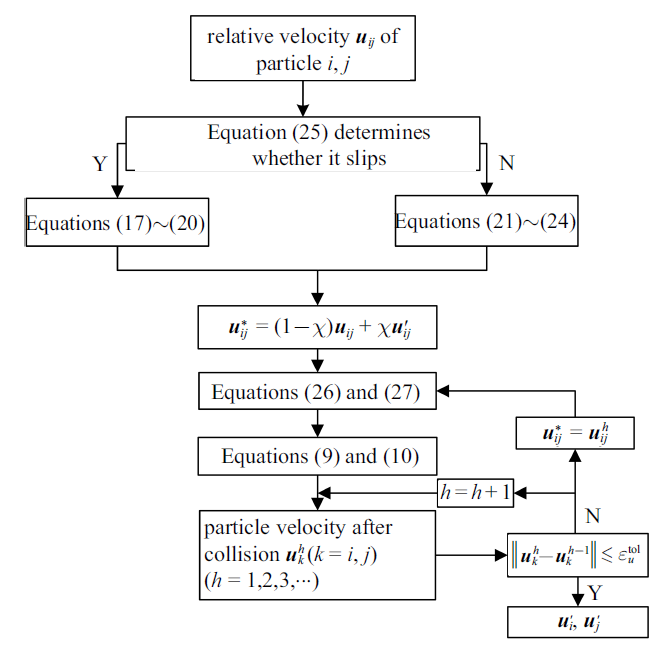Fig.4   Calculation flowchart of velocity for Particle collision

### 1.4 耦合分析计算方法

$\left\| {\alpha_{f, N} -\alpha_{f, 0} } \right\|\leqslant \varepsilon_{f, \alpha }^tol$
$\left\| {{ S}_{f, N} -{ S}_{f, 0} } \right\|\leqslant \varepsilon_{f, S}^tol$

CFD-DEM耦合分析时, 其耦合计算流程如图5所示, CFD计算采用SIMPLE算法求解流体域连续性方程和动量方程, 根据颗粒和流体的相对速度, 计算颗粒受到的流体作用力${ F}_f$, 传递给DEM求解器, 并启动DEM计算. DEM采用牛顿第二运动定律求解颗粒运动方程, 根据颗粒在$\Delta t_p$的运动轨迹, 搜索和判断颗粒碰撞, 若颗粒产生碰撞, 需修正颗粒计算时间步长. 根据颗粒在计算域内位置和受力的变化计算得到流体的体积分数$\alpha_f$和动量交换力源项${ S}_f$, 若不满足式(31)和式(32), 传递给CFD求解器, 自动更新流体计算时间步长, 并推进CFD计算.

### 图5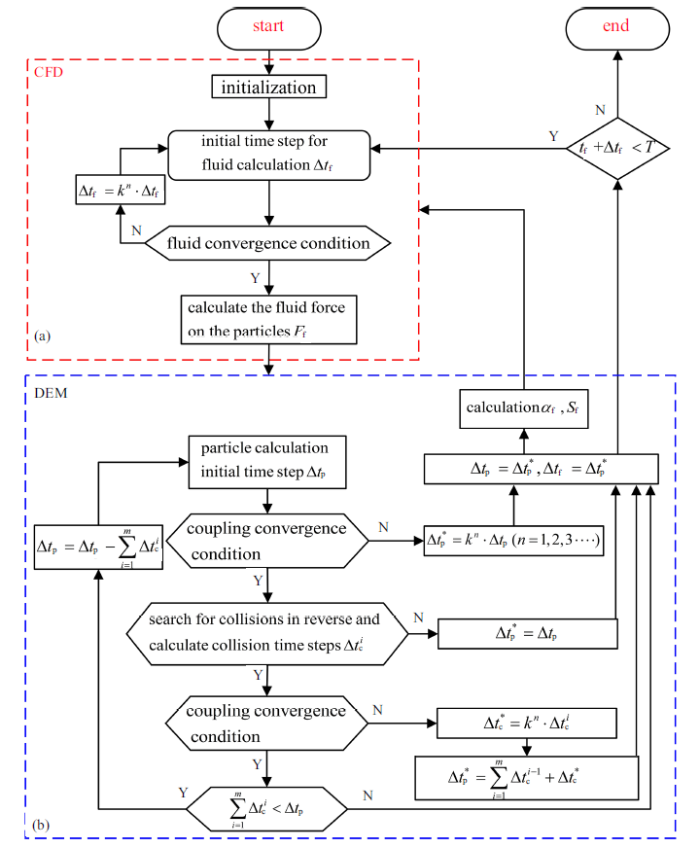Fig.5   Flow chart of CFD-DEM coupling calculation

CFD求解如图5(a)所示, 对流体计算域进行初始化, 设置流体初始计算时间步长$\Delta t_f$, 判断流体是否满足收敛条件, 若满足, CFD求解结束. 若不满足, 缩短流体时间步$\Delta t_f =k^{n}\cdot \Delta t_f$ $(k\leqslant 1$为修正系数, $n=1, 2, 3, \cdots$为流体时间步缩短次数), 重新进行CFD计算, 直至满足流体收敛条件, CFD求解结束.

CFD求解结束后, 计算$\Delta t_f$时刻颗粒受到的流体力${ F}_f$传递给DEM, 并启动DEM求解.

DEM求解如图5(b)所示, 设置颗粒计算初始时间步长$\Delta t_p =\Delta t_f$, 判断颗粒在$\Delta t_p$时刻计算结果是否满足式(31)、式(32)颗粒与流体耦合收敛条件, 若不满足, 缩短颗粒时间步$\Delta t_p^{\ast } =k^{n}\cdot \Delta t_p$, 重新进行DEM求解, 直至满足耦合收敛条件, DEM求解结束, 此时耦合分析的时间步为$\Delta t_p^{\ast }$. 若满足, 根据颗粒在$\Delta t_p$的运动轨迹, 搜索和判断颗粒是否产生碰撞, 若无碰撞, $\Delta t_p^{\ast } =\Delta t_p$, DEM求解结束. 若存在碰撞, 计算得到最先发生碰撞的时间$\Delta t_c^{i} \leqslant \Delta t_p$, 并根据$\Delta t_c^{1}$的颗粒位置, 判断是否满足耦合收敛条件. 若不满足, 缩短颗粒求解时间$\Delta t_c^{\ast } =k^{n}\cdot \Delta t_c^{i}$, 重新进行DEM求解, 直至满足耦合收敛条件, DEM求解结束, 此时耦合分析的时间步为$\Delta t_p^{\ast } =\sum_{i=1}^m {\Delta t_c^{i-1} } +\Delta t_c^{\ast }$(其中$i=$1时, 时间步内第1次搜索到碰撞, $\Delta t_p^{\ast } =\Delta t_c^{\ast } )$. 若满足, 需判断碰撞累计时长是否满足$\sum_{i=1}^m {\Delta t_c^{i} }<\Delta t_p$, 若不满足, DEM求解结束. 若满足, 则以剩余时间$\Delta t_p=\Delta t_p -\sum_{i=1}^m {\Delta t_c^{i} }$作为新的颗粒时间步长重新进行颗粒计算, 并搜索颗粒碰撞, 根据$\Delta t_c^{2}$的颗粒位置, 判断是否满足耦合收敛条件, 若不满足, 缩短颗粒求解时间$\Delta t_c^{\ast } =k^{n}\cdot \Delta t_c^{2}$, 重新进行DEM求解, 直至满足耦合收敛条件, DEM求解结束, 此时耦合分析的时间步为$\Delta t_p^{\ast } =\sum_{i=1}^m {\Delta t_c^{i-1} } +\Delta t_c^{\ast }$ (其中$i=$2时, 时间步内第2次搜索到碰撞, $\Delta t_p^{\ast } =\Delta t_c^{1} +\Delta t_c^{\ast } )$. 若满足耦合条件, 再次判断碰撞累计时长是否满足$\sum_{i=1}^m {\Delta t_c^{i} } <\Delta t_p (i=$2时, $\Delta t_c^{1} +\Delta t_c^{2} <\Delta t_p )$, 若不满足DEM求解结束. 若满足, 重新以剩余时间$\Delta t_p =\Delta t_p-\sum_{i=1}^m {\Delta t_c^{i} }$进行颗粒计算直至碰撞累计时长$\sum_{i=1}^m {\Delta t_c^{i} }<\Delta t_p$不成立, 或者不满足耦合收敛条件, 此时$\Delta t_p^{\ast }=\sum_{i=1}^m {\Delta t_c^{n-1} } +\Delta t_c^{\ast }$ (时间步内进行$n$次碰撞) DEM求解结束.

DEM求解结束后, 计算流体体积分数$\alpha_f$和动量交换力源项${ S}_f$, 并根据耦合分析时间步$\Delta t_p^{\ast }$, 对颗粒和流体计算时间步进行修正$\Delta t_p =\Delta t_p^{\ast } , \Delta t_f =\Delta t_p^{\ast }$, 判断流体计算时间$t_f +\Delta t_f<T$是否成立, 若成立, 将$\alpha_f$和${ S}_f$传递给CFD, 重新开始CFD求解; 若不成立, 则耦合计算结束.

## 2 颗粒碰撞搜索的逆向迭代算法

### 图6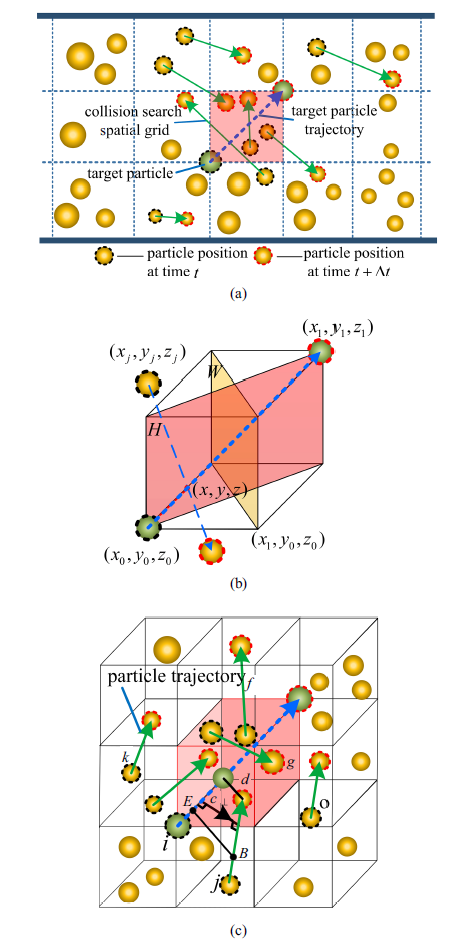Fig.6   Search diagram of particle collision: (a) Schematic diagram of variable grid establishment; (b) schematic diagram of particle trajectories passing through the spatial search grid; (c) schematic diagram of particle spacing screening

### 2.2 目标颗粒碰撞列表建立

(1)在计算时间步内, 筛选运动轨迹与空间网格体存在交集的颗粒, 如图6(a)所示.

$\left. {\begin{array}{l} x=x_{j} +\phi \cdot X_{j} \\ y=y_{j} +\phi \cdot Y_{j} \\ z=z_{j} +\phi \cdot Z_{j} \\ \end{array}} \right\}$

$H$平面的点法式方程为

$n_{1} \cdot (x-x_{0} )+n_{2} \cdot (y-y_{0} )+n_{3} \cdot (z-z_{0} )=0$

$\phi =\dfrac{(x_{0} -x_{j} )\cdot n_{1} +(y_{0} -y_{j} )\cdot n_{2} +(z_{0} -z_{j} )\cdot n_{3}}{n_{1} \cdot X_{j} +n_{2} \cdot Y_{j} +n_{3} \cdot Z_{j}}$

(2)依据颗粒与目标颗粒运动轨迹间距离小于或等于两颗粒半径之和的条件, 再次筛选可能碰撞颗粒, 如图6(c)所示.

${ e}\times { b}=\left| \begin{array}{*{20}c} i&j & k \\ {X_{i} }&{Y_{i} }& {Z_{i} } \\ {X_{j} }&{Y_{j} }&{Z_{j} } \\ \end{array} \right|=\langle i\left| \begin{array}{*{20}c} {Y_{i} }&{Z_{i} } \\ {Y_{j} }&{Z_{j} } \\ \end{array} \right|, \ \ -j\left| \begin{array}{*{20}c} {X_{i} }& {Z_{i} } \\ {X_{j} }& {Z_{j} } \\ \end{array} \right|, \\ k\left|\begin{array}{*{20}c} {X_{i} }& {Y_{i} } \\ {X_{j} }&{Y_{j} } \\ \end{array} \right| \rangle=(X, Y, Z)$

$d=\dfrac{\left| {{ e b}\times { c}_{\bot } } \right|}{\left| {{ c}_{\bot } } \right|}$

### 2.3 碰撞颗粒的逆向搜索算法

$\left. {\begin{array}{l} x_{i\left( {t+\Delta t_c } \right)} =x_{i\left( t \right)} +\Delta t_c \left[ {ku_{i\left( t \right)}^{x} +(1-k)u_{i\left( {t+\Delta t_c } \right)}^{x} } \right] \\ y_{i\left( {t+\Delta t_c } \right)} =y_{i\left( t \right)} +\Delta t_c \left[ {ku_{i\left( t \right)}^{y} +(1-k)u_{i\left( {t+\Delta t_c } \right)}^{y} } \right] \\ z_{i\left( {t+\Delta t_c } \right)} =z_{i\left( t \right)} +\Delta t_c \left[ {ku_{i\left( t \right)}^{z} +(1-k)u_{i\left( {t+\Delta t_c } \right)}^{z} } \right] \\ \end{array}} \right\}$
$\left. {\begin{array}{l} x_{j\left( {t+\Delta t_c } \right)} =x_{j\left( t \right)} +\Delta t_c \left[ {ku_{j\left( t \right)}^{x} +(1-k)u_{j\left( {t+\Delta t_c } \right)}^{x} } \right] \\ y_{j\left( {t+\Delta t_c } \right)} =y_{j\left( t \right)} +\Delta t_c \left[ {ku_{j\left( t \right)}^{y} +(1-k)u_{j\left( {t+\Delta t_c } \right)}^{y} } \right] \\ z_{j\left( {t+\Delta t_c } \right)} =z_{j\left( t \right)} +\Delta t_c \left[ {ku_{i\left( t \right)}^{z} +(1-k)u_{i\left( {t+\Delta t_c } \right)}^{z} } \right] \\ \end{array}} \right\}$

### 图7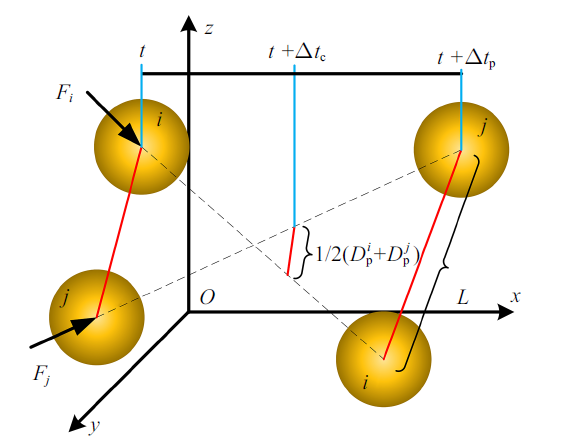Fig.7   Schematic diagram of particle collision time solution

$\Big[\left( {x_{i\left( {t+\Delta t_c } \right)} -x_{j\left( {t+\Delta t_c } \right)} } \right)^{2}+\left( {y_{i\left( {t+\Delta t_c } \right)} -y_{j\left( {t+\Delta t_c } \right)} } \right)^{2}+ \\ \left( {z_{i\left( {t+\Delta t_c } \right)} -z_{j\left( {t+\Delta t_c } \right)} } \right)^{2}\Big]^{1/2} =\dfrac{1}{2}\left( {D_p^{i} +D_p^{j} } \right)$

$\Delta t_c^{4}+A\Delta t_c^{3}+B\Delta t_c^{2}+C\Delta t_c +D=0\left( {0<\Delta t_c <\Delta t_p } \right)$
$\left. \begin{array}{l} A=2\bigg[ \left( {x_{i\left( t \right)} -x_{j\left( t \right)} } \right)\left( {a_{i\left( t \right)}^{x} -a_{j\left( t \right)}^{x} } \right)+\left( {y_{i\left( t \right)} -y_{j\left( t \right)} } \right)\cdot\\[2.5mm]\qquad \left( {a_{i\left( t \right)}^{y} -a_{j\left( t \right)}^{y} } \right) +\left( {z_{i\left( t \right)} -z_{j\left( t \right)} } \right)\left( {a_{i\left( t \right)}^{z} -a_{j\left( t \right)}^{z} } \right) \bigg]\bigg/\\[2.5mm]\qquad \Big( {a_{i\left( t \right)}^{x} -a_{j\left( t \right)}^{x} } + {a_{i\left( t \right)}^{y} -a_{j\left( t \right)}^{y} } + {a_{i\left( t \right)}^{z} -a_{i\left( t \right)}^{z} }\Big) \\[2.5mm] B=\bigg[ \left( {u_{i\left( t \right)}^{x} -u_{j\left( t \right)}^{x} } \right)^{2}+\left( {u_{i\left( t \right)}^{y} -u_{j\left( t \right)}^{y} } \right)^{2}+\left( {u_{i\left( t \right)}^{z} -u_{j\left( t \right)}^{z} } \right)^{2}+\\[2.5mm]\qquad 2\left( {x_{i\left( t \right)} -x_{j\left( t \right)} } \right)\left( {a_{i\left( t \right)}^{x} -a_{j\left( t \right)}^{x} } \right)+2\left( {y_{i\left( t \right)} -y_{j\left( t \right)} } \right)\cdot\\[2.5mm]\qquad \left( {a_{i\left( t \right)}^{y} -a_{j\left( t \right)}^{y} } \right) +2\left( {z_{i\left( t \right)} -z_{j\left( t \right)} } \right)\left( {a_{i\left( t \right)}^{z} -a_{j\left( t \right)}^{z} } \right) \bigg]\bigg/\\[2.5mm]\qquad \Big( {a_{i\left( t \right)}^{x} -a_{j\left( t \right)}^{x} } + {a_{i\left( t \right)}^{y} -a_{j\left( t \right)}^{y} } + {a_{i\left( t \right)}^{z} -a_{i\left( t \right)}^{z} } \ \Big)\\[2.5mm] C=\bigg[ 2\left( {u_{i\left( t \right)}^{x} -u_{j\left( t \right)}^{x} } \right)\left( {x_{i\left( t \right)} -x_{j\left( t \right)} } \right)+2\left( {u_{i\left( t \right)}^{y} -u_{j\left( t \right)}^{y} } \right)\cdot\\[2.5mm]\qquad \left( {y_{i\left( t \right)} -y_{j\left( t \right)} } \right) +2\left( {u_{i\left( t \right)}^{z} -u_{j\left( t \right)}^{z} } \right)\left( {z_{i\left( t \right)} -z_{j\left( t \right)} } \right) \bigg]\bigg/\\[2.5mm]\qquad\Big( {a_{i\left( t \right)}^{x} -a_{j\left( t \right)}^{x} } + {a_{i\left( t \right)}^{y} -a_{j\left( t \right)}^{y} } + {a_{i\left( t \right)}^{z} -a_{i\left( t \right)}^{z} } \Big) \\[2.5mm] D=\bigg\{\bigg[ \left( {x_{i\left( t \right)} -x_{j\left( t \right)} } \right)^{2}+\left( {y_{i\left( t \right)} -y_{j\left( t \right)} } \right)^{2}+\left( {z_{i\left( t \right)} -z_{j\left( t \right)} } \right)^{2} \bigg]-\\[2.5mm]\qquad \dfrac{1}{4}\left( {D_p^{i} +D_p^{j} } \right)^{2}\bigg\}\bigg/\Big( {a_{i\left( t \right)}^{x} -a_{j\left( t \right)}^{x} } + {a_{i\left( t \right)}^{y} -a_{j\left( t \right)}^{y} }+\\[2.5mm]\qquad a_{i\left( t \right)}^{z} -a_{i\left( t \right)}^{z} \Big)\\ \end{array} \right\}$

### 图8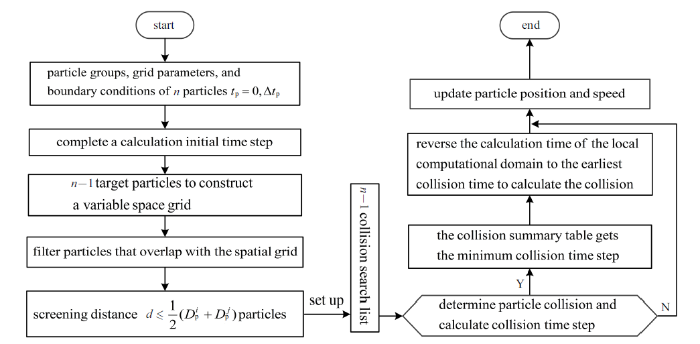Fig.8   Particle swarm collision search solution process

## 3 CFD-DEM耦合分析及颗粒碰撞搜索算法验证

### 图9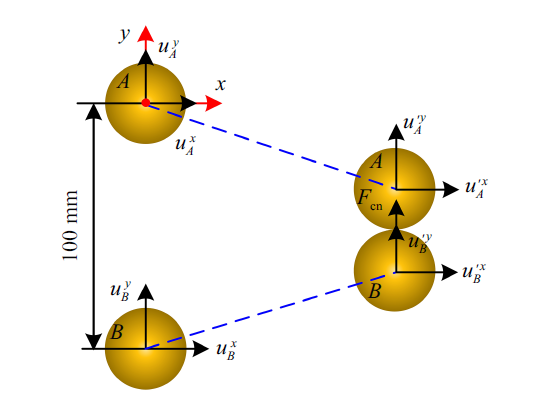Fig.9   Schematic diagram of particle position

Table 1  Theoretical and numerical simulation results of movement and collision of two particles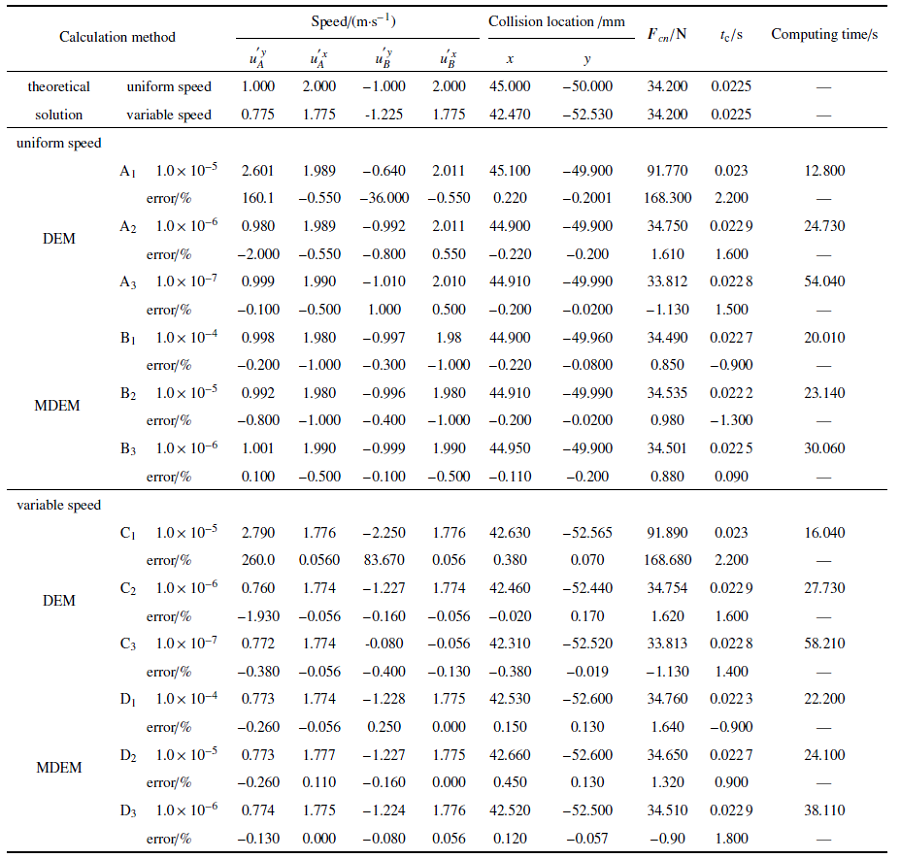DEM和MDEM取计算时间0.1 s, 计算时间步为10$^{-4}$ s, 10$^{-5}$ s, 10$^{-6}$ s, 10$^{-7}$ s对颗粒匀速、变速运动进行数值模拟, 得到碰撞力、碰撞速度、碰撞位置等结果一并列入表1.模拟所用计算机为英特尔 Corei7-9700 @3.00 GHz 八核, 主显卡AMD Radeon RX 550 Series, 内存16 GB.

### 图10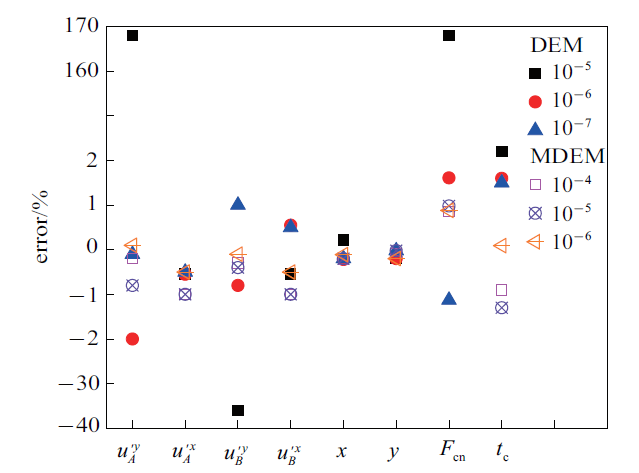Fig.10   Error analysis diagram of two particles in uniform motion

### 图11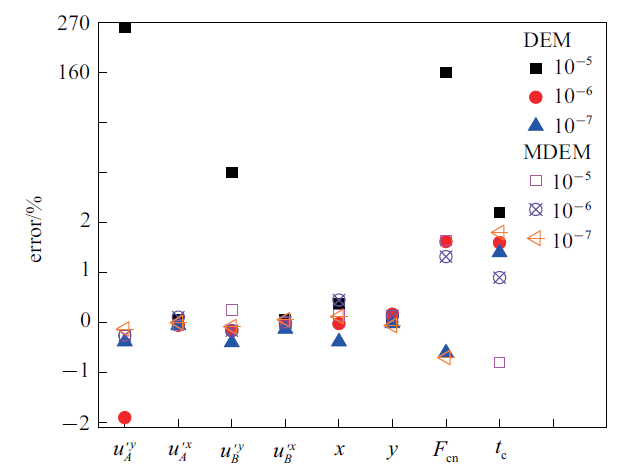Fig.11   Error analysis diagram of variable speed movement of two particles

### 图12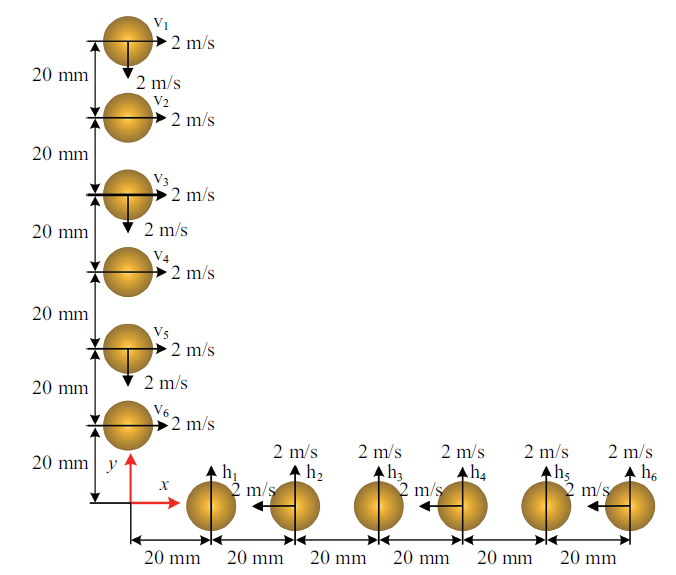Fig.12   Schematic diagram of initial position of multi-particle collision

Table 2  Numerical simulation results of multi-particle motion and collision (DEM)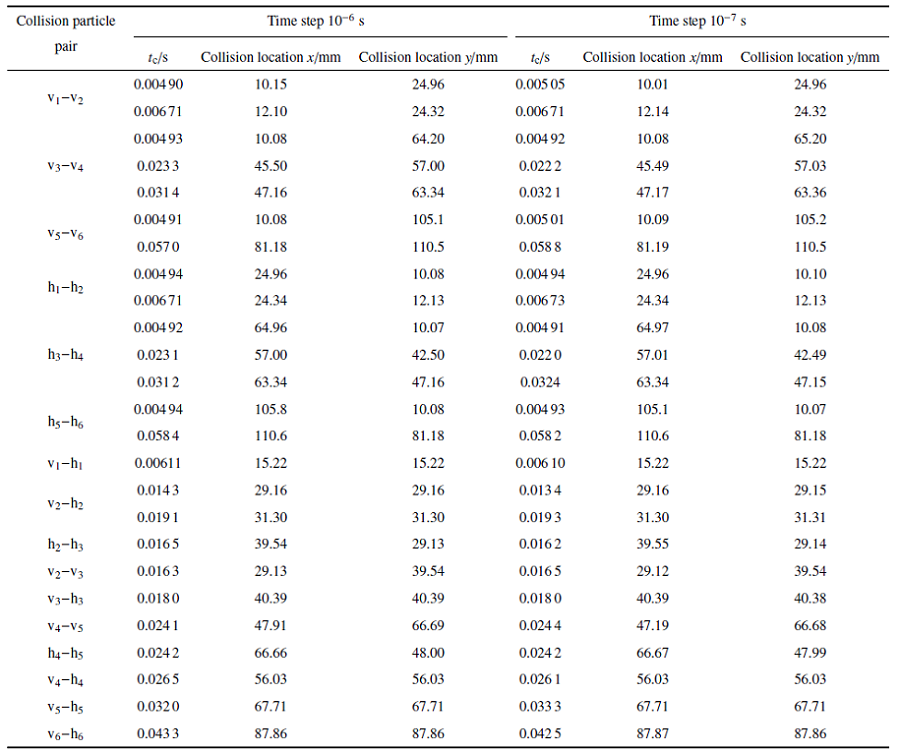### 图13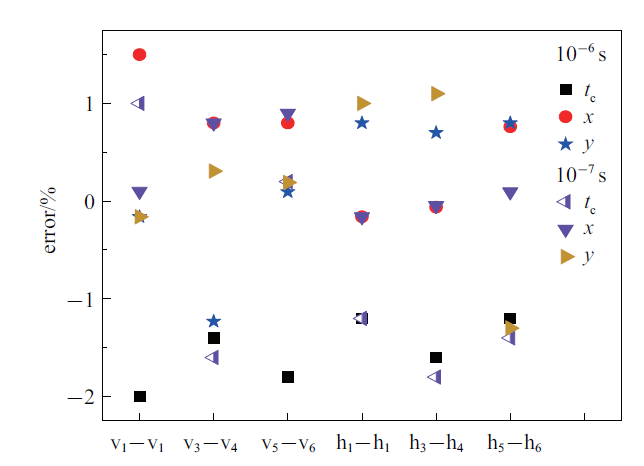Fig.13   DEM particle collision time and position error analysis diagram

Table 3  Numerical simulation results of multi-particle motion and collision (MDEM)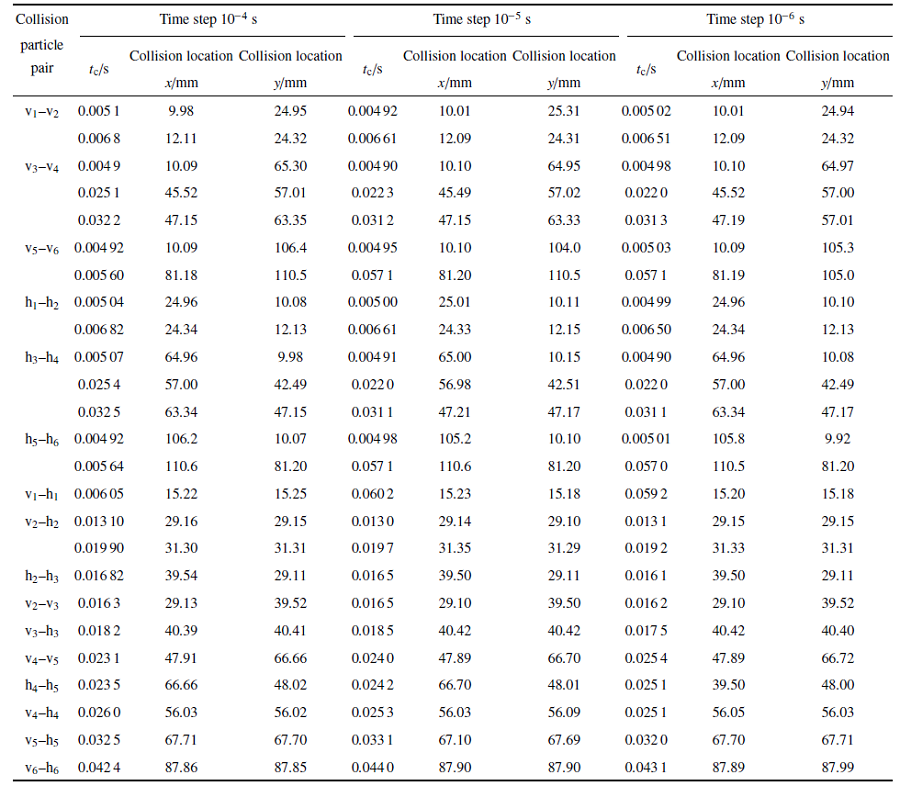### 图14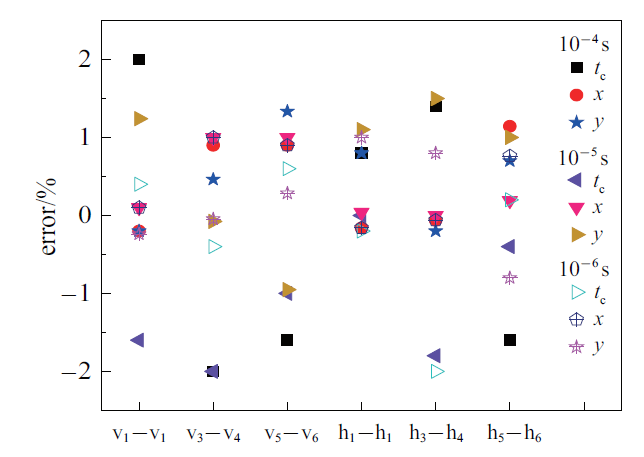Fig.14   MDEM particle collision time and position error analysis diagram

### 图15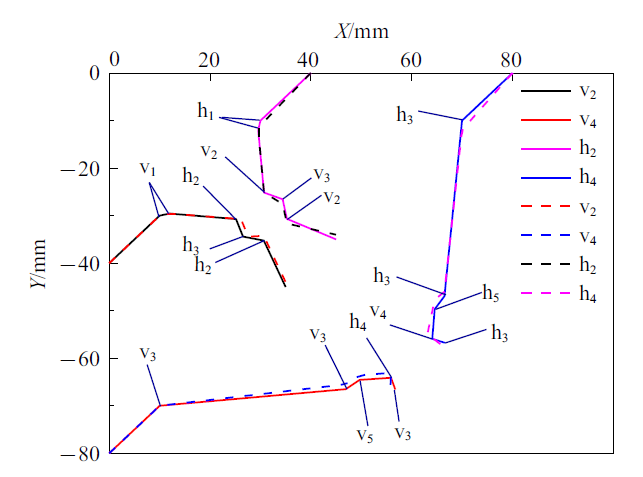Fig.15   Movement trajectories and collisions of more than one particle

### 3.3 CFD-DEM耦合分析算例

Table 4  Time step setting of particle-fluid coupling analysis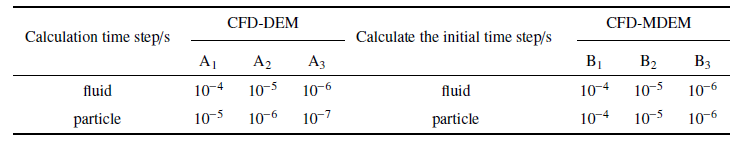CFD-DEM计算结果表明: 在A$_{1}$, A$_{2}$, A$_{3}$计算时间步得到颗粒碰撞次数分别为18次、22次、22次, 与算例3.2相比碰撞次数减少了3次, 原因在于流体的阻力使颗粒做减速运动, 计算耗时分别为18 min, 27 min, 42 min, 只有在颗粒计算时间步小于等于10$^{-6}$ s时, CFD-DEM耦合计算才能得到精确值.

CFD-MDEM计算结果表明: 在B$_{1}$, B$_{2}$, B$_{3}$计算时间步, 得到的颗粒碰撞次数均为22次, 计算耗时分别为22.5 min, 24 min, 31 min, 颗粒计算初始时间步的改变不影响CFD-MDEM耦合计算精度.

A$_{2}$流体计算时间步比B$_{3}$流体计算初始时间步大一个量级, A$_{2}$流体求解器启动10$^{4}$次, 颗粒求解器启动10$^{5}$次, B$_{3}$流体与颗粒求解器均启动100 383次. B$_{3}$比A$_{2}$多启动383次, 是由于MDEM算法根据耦合收敛条件, 修正计算初始时间步, 得到真实计算时间步比计算初始时间步小, B$_{3}$比A$_{2}$计算多耗时4 min. B$_{2}$流体计算初始时间步与A$_{2}$流体计算时间步相等, B$_{2}$颗粒计算初始时间步比A$_{2}$颗粒计算时间步大一个量级, B$_{2}$流体与颗粒求解器启均动11 479次, 与A$_{2}$流体求解次数相比求解器多启动1479次, 与B$_{3}$相比求解器多启动次数由383次增加到1479次, 是由于颗粒计算初始时间步的扩大, 颗粒求解不满足收敛条件次数增多造成. B$_{2}$比A$_{2}$计算少耗时3 min, 降低11.1%. B$_{1}$流体计算初始时间步比A$_{2}$流体计算时间步大一个量级, 颗粒计算初始时间步比A$_{2}$颗粒计算时间步大两个量级, B$_{1}$流体与颗粒求解器均启动3254次, 比A$_{2}$计算少耗时4.5 s, 降低16.7%. A$_{2}$和B$_{1}$颗粒h$_{2}$, h$_{3}$, h$_{4}$和v$_{2}$, v$_{4}$, v$_{6}$的运动轨迹基本一致, 如图16所示.

### 图16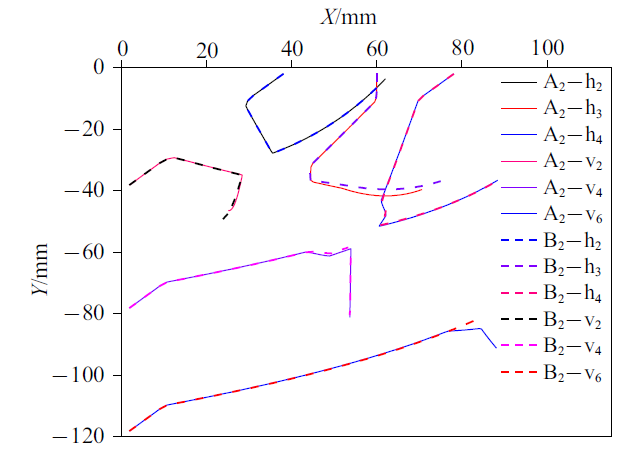Fig.16   Multiple particle trajectories in fluid

## 4 结论

(1)提出了颗粒碰撞搜索的改进算法, 以目标颗粒运动位移构建可变空间搜索网格, 筛选可能碰撞颗粒建立搜索列表, 减少了颗粒碰撞的搜索时间;采用逆向搜索方式判断颗粒碰撞, 避免了时间步选取过大对颗粒碰撞漏判, 确保了颗粒碰撞的准确描述. 通过两个颗粒和多个颗粒的运动和碰撞算例表明, 本方法计算时间步选取的大小对颗粒碰撞计算精度影响不大;两个颗粒在匀速和变速工况下的数值模拟计算耗时比DEM方法降低了19.1%和19.9%, 多个颗粒的数值模拟计算耗时比DEM方法降低了14.9%.

(2)建立了颗粒与流体计算时间步自动调整的耦合算法, 颗粒与流体计算初始时间步选取可以相同, 根据颗粒推进时间实时调整流体计算时间, 实现流体计算时间步随颗粒计算时间自动调整, 提高了颗粒与流体耦合的计算效率. 多个颗粒与流体耦合算例的数值模拟表明, 该算法的计算精度受颗粒和流体的初始计算时间步长影响较小, 可通过较大的颗粒时间来提高计算效率, 该算法在求解算例的耗时比CFD-DEM方法降低了16.7%.

## 参考文献 原文顺序 文献年度倒序 文中引用次数倒序 被引期刊影响因子

Saini N, Kleinstreuer C.

A new collision model for ellipsoidal particles in shear flow

Journal of Computational Physics, 2019, 376: 1028-1050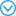All natural and a growing number of manufactured solid particles are non-spherical. Interesting fluid-particle dynamics applications include the transport of granular material, piling of seeds or grains, inhalation of toxic aerosols, use of nanofluids for enhanced cooling or improved lubrication, and optimal drug-targeting of tumors. A popular approach for computer simulations of such scenarios is the multi-sphere (MS) method, where any non-spherical particle is represented by an assemblage of spheres. However, the MS approach may lead to multiple sphere-to-sphere contact points during collision, and subsequently to erroneous particle transport and deposition. In cases where non-spherical particles can be approximated as ellipsoids with arbitrary aspect ratios, a new theory for particle transport, collision and wall interaction is presented which is more accurate computationally and more efficient than the MS method. In general, with the new ellipsoidal particle interaction (EPI) model, contact points and planes of ellipsoids, rather than spheres, are obtained based on a geometric potential algorithm. Then, interaction forces and torques of the colliding particles are determined via inscribed 'pseudo-spheres', employing the soft-particle approach. The off-center forces and moments are then transferred to the mass center of the ellipsoids to solve the appropriate translatory and angular equations of motion. Considering ellipses to illustrate the workings and predictive power of the new collision model, turbulent fluid-particle flow with the EPI model in a 2-D channel is simulated and compared with 3-D numerical benchmark results which relied on the MS method. The 2-D concentrations of micron particles with different aspect ratios matched closely with the 3-D cases. However, interesting differences occurred when comparing the particle-velocity profiles for which the 2-D EPI model generated somewhat larger particle velocities due to out-of-plane collisions, slightly higher particle-wall interactions, and two-way coupling effects. (C) Published by Elsevier Inc.(Liu Cheng, Shen Yongming.

Numerical simulation of two-phase movement of water and sand in a fixed bed curve

Chinese Journal of Theoretical and Applied Mechanics, 2009, 41(3): 318-328 (in Chinese))

Ge L, Evans GM, Moreno-Atanasio R.

CFD-DEM investigation of the interaction between a particle swarm and a stationary bubble: Particle-bubble collision efficiency

Powder Technology, 2020, 366: 641-652

Tanaka T, Tsuji M.

Numerical simulation of gas-solid two-phase flow in a vertical pipe: On the effect of inter-particle collision

ASME/FED Gas-Solid Flows, 1991, 121: 123-128

Acmae El Y, Xu S, Wang J.

A New method for computing particle collisions in Navier-Stokes flows

Journal of Computational Physics, 2019, 99: 108919

(Liu Xiangjun, Shi Lei, Xu Xuchang.

Research status of the Euler-Lagrangian method for dense gas-solid two-phase flow

Chinese Journal Computational Mechanics, 2007, 24(2): 166-172 (in Chinese))

Chen X, Wang J.

A comparison of two-fluid model, dense discrete particle model and CFD-DEM method for modeling impinging gas-solid flows

Powder Technology, 2014, 254: 94-102

GidaGidaspow D. Multiphase Flows and Fluidization. San Diego: Academic Press Inc, 1994

(Fu Xudong, Wang Guangqian.

The particle phase kinetic model of low-concentration solid-liquid two-phase flow

Acta Mechanic Sinica, 2003, 35(6): 650-659 (in Chinese))

(Liu Anyuan, Liu Shi.

Theoretical study on collision and heat transfer of particles in a fluidized bed

Proceedings of the CSEE, 2003, 23(3): 161-165 (in Chinese))

(Liu Xiangjun, Xu Xuchang.

Numerical simulation of dense gas-solid two-phase flow in circulating fluidized bed

Proceedings of the CSEE, 2003, 23(5): 162-166 (in Chinese))

(Sun Ping, Fang Jianren, Xia Zhenhai, et al.

Model and verification of turbulent gas-solid two-phase flow considering collisions between particles

Progress in Natural Science, 1998, 5(8): 572-580 (in Chinese))

Hockney RW, Eastwood JW.

Computer simulation using particles

Institute of Physics, 1988, 76: 249-256

Baraff D.

Interactive simulation of solid rigid bodies

IEEE Computer Graphics & Applications, 1995, 15(3): 63-75

Schaefer BC, Quigley SF, Chan AHC.

Acceleration of the discrete element method (DEM) on a reconfigurable co-processor

Computers & Structures, 2004, 82(21): 1707-1718

Allen M, Tildesley D. Computer Simulation of Liquids. Oxford: Clarendon Press, 1987

Vemuri BC, Chen L, Waltin O, et al.

Efficient and accurate collision detection for granular flow simulation

Graphical Models & Image Processing, 1998, 60(6): 403-422

Li CF, Feng YT, Owen DRJ.

SMB: Collision detection based on temporal coherence

Computer Methods in Applied Mechanics & Engineering, 2006, 195(22): 2252-2269

Sigurgeirsson H, Stuart A, Wan W.

Algorithms for particle-field simulations with collisions

Journal of Computational Physics, 2001, 172: 766-807

Yao LM, Xiao ZM, Liu JB, et al.

An optimized CFD-DEM method for fluid-particle coupling dynamics analysis

International Journal of Mechanical ences, 2020, 174: 105503

Banaei M, Jegers J, van Sint Annaland J.

Tracking of particles using TFM in gas-solid fluidized beds

Advanced Powder Technology, 2018, 29(10): 2538-2547

Sharma K, Mallick SS, Mittal A.

A study of energy loss due to particle to particle and wall collisions during fluidized dense-phase pneumatic transport

Powder Technology, 2019, 362: 707-716

Ariane B, Gregory S.

Experimental methods in chemical engineering: Unresolved CFD-DEM

Canadian Journal of Chemical Engineering, 2020, 98(2): 424-440

Auton TR, Hunt JCR, Prud'Homme M.

The force exerted on a body in inviscid unsteady non-uniform rotational flow

J Fluids Mech, 1988, 197: 241-257

Eskin D, Ratulowski J, Akbarzadeh K.

Modeling of particle deposition in a vertical turbulent pipe flow at a reduced probability of particle sticking to the wall

Chemical Engineeringence, 2011, 66(20): 4561-4572

Liu RJ, Xiao R, Ye M, et al.

Analysis of particle rotation in fluidized bed by use of discrete particle model

Advanced Powder Technology, 2018, 29(7): 1655-1663

Thomas PJ.

On the influence of the Basset history force on the motion of a particle through a fluid

Physics of Fluids A Fluid Dynamics, 1992, 4(9): 2090-2093

Cheng J, Dou Y, Zhang N, et al.

A new method for predicting erosion damage of suddenly contracted pipe impacted by particle cluster via CFD-DEM

Materials, 2018, 11(10): 1858-1869

Zhang Y.

Application and improvement of computational fluid dynamics (CFD) in solid particle erosion modeling. [PhD Thesis]

The University of Tulsa, 2006

Salmana AD, Gorhamb DA, Szabó M, et al.

Spherical particle movement in dilute pneumatic conveying

Powder Technology, 2005, 153: 43-50(Fan Fengxian, Wang Zhiqiang, Liu Ju, et al.

Discrete element simulation of particle capillary effect in vertical vibrating tube

Chinese Journal of Theoretical and Applied Mechanics, 2019, 51(2): 415-424 (in Chinese))(Li Hongjing, Mei Yuchen, Ren Yongliang.

An integral differential method for structural dynamic time history analysis

Chinese Journal of Theoretical and Applied Mechanics, 2019, 51(5): 1507-1516 (in Chinese))(Wei Xinrong, Duan Shaozhen, Sun Jinlong, et al.

Particle velocity prediction of slope rolling rock based on impact model

Chinese Journal of Theoretical and Applied Mechanics, 2020, 52(3): 707-715 (in Chinese))

(Xu Zhilun. Concise Course of Elasticity. Beijing: Higher Education Press, 2002 (in Chinese))(Wang Shuai, Hao Zhenhua, Xu Pengfei, et al.

Coarse particle kinetics and numerical simulation of dense gas-solid two-phase flow

Chinese Journal of Theoretical and Applied Mechanics, 2012, 44(2): 278-286 (in Chinese))

Alobaid F, Baraki N, Epple B.

Investigation into improving the efficiency and accuracy of CFD/DEM simulations

Particuology, 2014, 16(5): 41-53

/

 〈〉